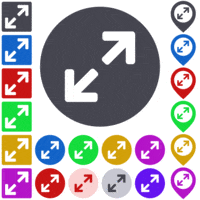# Math Worksheets Land

Math Worksheets For All Ages

# Math Worksheets Land

Math Worksheets For All Ages

Home > Topics >

# Greater Than, Less Than, Or Equal Worksheets

As students begin to develop a sense of numeracy it starts with understanding that one number is different from another. We are now at a place where we want to actual put the label on that relationship that exists. If one value is larger than the other, we say that larger value is greater than the other one. We symbolize greater with the right-hand open symbol (>). The smaller value has a less than relationship and we symbolize that with the left-hand open symbol (<). There are a number of different ways to remember how to compare values. If you have an unequal relationship, you always point the arrow to the smaller value. You can also use the alligator model and say that the alligator is always going to eat the larger meal (value). This means that his jaws (the open part) will always point at the bigger value. This collection of worksheets will help identify the relationships between values and show you how to label them. These are also know as math comparison worksheets. We try to cover all that common comparisons.

### Tips for Teaching the Concepts of Greater Than, Less Than, Or EqualMany mathematical representative terms are difficult for students, and they find them tricky to remember. Just like greater than, less than and equal to. But don’t worry, these concepts are fairly simple and with the right example, your students will be able to recognize what greater than, less than, and equal is.

This particular concept of comparison is a pivotal concept that lays the foundation for a great number of concepts. So, it is paramount that students understand this idea so that they can apply it going forward. This is the key feature of an inequality, and it is important to form a good mindset about it. Here are some ideas that we encourage you to try with your students:

Greater than - Pick two students from your class. Give four books to one of them and two to the other. Now, tell them the difference between the quantities. Explain what does greater mean and lesser mean. Now ask them to identify which student has the larger quantity. Once they have done that, tell them how to compare, how quantities are different from one another. Once their concepts have clarified, now tell them that Student A has a greater number of books than Student B.

Less than - Once they have identified the greater than concept, the rest becomes easy. All you have to do now is point them in the right direction. Just ask them if we have to highlight the lesser quantity, what do we do. They will get the idea and say that Student B has lesser books than Student A.

Equal - Now, give two more books to student B. Now, start telling your students what it means when two quantities are the same. Whenever two quantities, there is no comparison left and instead, we use the term equal. So, the sentence will be read as Student A, and Student B have an equal number of books.

Unlock all the answers, worksheets, homework, tests and more!
Save Tons of Time! Make My Life Easier Now

## Thanks and Don't Forget To Tell Your Friends!

I would appreciate everyone letting me know if you find any errors. I'm getting a little older these days and my eyes are going. Please contact me, to let me know. I'll fix it ASAP.